A Survey of Gaussian Convolution Algorithms
DCT-based Gaussian convolution

Convolution via multiplication in the DCT domain. More...

## Detailed Description

Convolution via multiplication in the DCT domain.

Via the convolution-multiplication property, discrete cosine transforms (DCTs) are an effective way to implement Gaussian convolution. We follow Martucci's use of DCTs to perform convolution with half-sample symmetric boundary handling,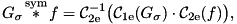where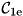and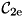denote respectively the DCT-I and DCT-II transforms of the same period length. This DCT-based convolution is equivalent to (but is faster and uses less memory) than FFT-based convolution with the symmetrized signal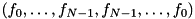.

The FFTW library is used to compute the DCT transforms.

The process to use these functions is the following:

1. dct_precomp() or dct_precomp_image() to set up the convolution
2. dct_gaussian_conv() to perform the convolution itself (it may be called multiple times if desired)
3. dct_free() to clean up
Example
Reference
• S. Martucci, "Symmetric convolution and the discrete sine and cosine transforms," IEEE Transactions on Signal Processing SP-42, pp. 1038-1051, 1994. http://dx.doi.org/10.1109/78.295213

## Data Structures

struct  dct_coeffs_
FFTW plans and coefficients for DCT-based Gaussian convolution. More...

## Typedefs

typedef struct dct_coeffs_ dct_coeffs
FFTW plans and coefficients for DCT-based Gaussian convolution.

## Functions

int dct_precomp (dct_coeffs *c, num *dest, const num *src, long N, long stride, double sigma)
DCT precomputations for Gaussian convolution. More...

int dct_precomp_image (dct_coeffs *c, num *dest, const num *src, int width, int height, int num_channels, double sigma)
DCT precomputations for 2D Gaussian convolution. More...

void dct_free (dct_coeffs *c)
Release FFTW plans associated with a dct_coeffs struct. More...

void dct_gaussian_conv (dct_coeffs c)
Perform DCT-based Gaussian convolution. More...

## Function Documentation

 void dct_free ( dct_coeffs * c )

Release FFTW plans associated with a dct_coeffs struct.

Parameters
 c dct_coeffs created by dct_precomp() or dct_precomp_image()

Definition at line 203 of file gaussian_conv_dct.c.

 void dct_gaussian_conv ( dct_coeffs c )

Perform DCT-based Gaussian convolution.

Parameters
 c dct_coeffs created by dct_precomp() or dct_precomp_image()

This routine performs 1D and 2D Gaussian convolution with symmetric boundary handling using DCT transforms,whereanddenote respectively the DCT-I and DCT-II transforms of the same period length.

In one dimension, the DCT-I transform of the Gaussian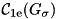is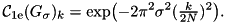The DCT-II transforms of the signal are performed using the FFTW library, and the plans are prepared by dct_precomp() or dct_precomp_image().

Definition at line 156 of file gaussian_conv_dct.c.

 int dct_precomp ( dct_coeffs * c, num * dest, const num * src, long N, long stride, double sigma )

DCT precomputations for Gaussian convolution.

Parameters
 c dct_coeffs pointer to hold precomputations dest output convolved data src data to be convolved, modified in-place if src = dest N number of samples stride stride between successive samples sigma standard deviation of the Gaussian in pixels
Returns
1 on success, 0 on failure

This routine performs precomputations for 1D DCT-based Gaussian convolution, to be used in dct_gaussian_conv(). FFTW transform plans are prepared for the forward and inverse DCT-II transform and the value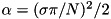is precomputed.

The convolution can be performed in-place by setting src = dest (the source array is overwritten with the result).

Note
For use of the num typedef with FFTW's APIs, the macro FFT(functionname) expands to fftw_functionname if num is double or fftwf_functionname if num is float.

Definition at line 53 of file gaussian_conv_dct.c.

 int dct_precomp_image ( dct_coeffs * c, num * dest, const num * src, int width, int height, int num_channels, double sigma )

DCT precomputations for 2D Gaussian convolution.

Parameters
 c dct_coeffs pointer to hold precomputations dest output convolved image src input image, modified in-place if src = dest width image width height image height num_channels number of image channels sigma standard deviation of the Gaussian in pixels
Returns
1 on success, 0 on failure

Similar to dct_precomp(), this routine performs precomputations for 2D DCT-based Gaussian convolution, to be used in dct_gaussian_conv().

The convolution can be performed in-place by setting src = dest (the source array is overwritten with the result).

Definition at line 100 of file gaussian_conv_dct.c.